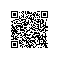# 从零开始学_JavaScript_系列（20）——js系列&lt;7&gt;（函数原型的两种声明方式、函数的作用域）

（20）函数的原型

①Object.create(obj)

var obj = {
a: 1
}
var o1 = Object.create(obj);
console.log(obj);
console.log(o1);

Object {a: 1}

Object {}

②使用构造函数方法：

①和②之间的区别：

var obj = {
a: 1
}
var o1 = Object.create(obj);
var o2 = Object.create(o1);
o1.b = 5;
o2.c = 2;
console.log(o1.b);
console.log(o2.b);

o1和o2都有属性b，并且值为5；

var obj = function () {
a: 1
}
var o1 = function(){}
o1.prototype = new obj();
var o2 = function(){}
o2.prototype = new o1();
o1.b = 5;
o2.c = 2;
console.log(o1.b);
console.log(o2.b);

var obj = {
a: 1
}
var o1 = Object.create(obj);
var o2 = Object.create(o1);
o1.b = 3;
console.log(o2.b);
o1.b = 5;
console.log(o2.b);

④hasOwnProperty()

var obj = {
a: 1
}
var o1 = Object.create(obj);
var o2 = Object.create(o1);
o1.b = 5;
console.log(o2.hasOwnProperty("b"));
o2.b = 2;
console.log(o2.hasOwnProperty("b"));

o2.prototype.b = 5;

（21）函数的作用域

①静态作用域：（JS使用的是这种）

var x = 1;
function a() {
console.log(x);
}
function b() {
var x = 2;
a();
}
b();

var x = 1;
function b() {
var x = 2;
function a() {
console.log(x);
}
a();
}
b();

②JS变量的作用域：全局作用域、函数作用域。没有块级作用域（即{}之间声明的变量的作用域）；

③with方法的临时作用域：

var x = 1;
with ({x: 2}) {
function a() {
console.log(x);
}
(function () {
console.log(" " + x);   //输出2
a() //输出1
})();
}
console.log(x); //输出1使用钉钉扫一扫加入圈子
+ 订阅